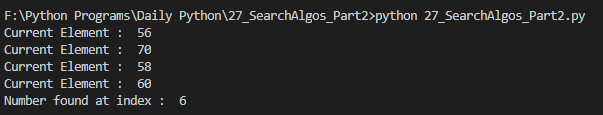# Implementing Fibonacci Search algorithm in Python| Daily Python #27

This article is a tutorial on implementing the Fibonacci Search algorithm in Python and is in continuation with Daily Python #21

This article is a part of Daily Python challenge that I have taken up for myself. I will be writing short python articles daily.

If you haven’t read Part 1 of basic searching algorithms then you can read it from here:

# Fibonacci Search

Fibonacci Search is a comparison-based technique that uses Fibonacci numbers to search an element in a sorted array.

1. Works for sorted arrays
2. A Divide and Conquer Algorithm.
3. Has Log n time complexity.

## Differences with Binary Search:

1. Fibonacci Search divides given array in unequal parts
2. Binary Search uses the division operator to divide range. Fibonacci Search doesn’t use /, but uses + and -. The division operator may be costly on some CPUs.
3. Fibonacci Search examines relatively closer elements in subsequent steps. So when the input array is big that cannot fit in CPU cache or even in RAM, Fibonacci Search can be useful.

## Background:

Fibonacci Numbers are recursively defined as F(n) = F(n-1) + F(n-2), F(0) = 0, F(1) = 1. First few Fibonacci Numbers are 0, 1, 1, 2, 3, 5, 8, 13, 21, 34, 55, 89, 144, …

# Let’s define a function to generate a Fibonacci number at the index of n

`def FibonacciGenerator(n):    if n < 1:        return 0    elif n == 1:        return 1    else:        return FibonacciGenerator(n - 1) + FibonacciGenerator(n - 2)`

# Let’s consider the following array and the number to be searched

`arr = [10, 22, 30, 44, 56, 58, 60, 70, 100, 110, 130] x = 60`

# Now, let’s define a function that will divide the list and search for the number as per the Fibonacci Search

`def FibonacciSearch(arr, x):    m = 0     while FibonacciGenerator(m) < len(arr): #         m = m + 1     offset = -1        while (FibonacciGenerator(m) > 1):        i = min( offset + FibonacciGenerator(m - 2) , len(arr) - 1)        print('Current Element : ',arr[i])        if (x > arr[i]):            m = m - 1            offset = i        elif (x < arr[i]):            m = m - 2        else:            return i            if(FibonacciGenerator(m - 1) and arr[offset + 1] == x):        return offset + 1    return -1print('Number found at index : ',FibonacciSearch(arr, x))`Snip of the output of the above code snippet

I hope this article was helpful, do leave some claps if you liked it.

--

--